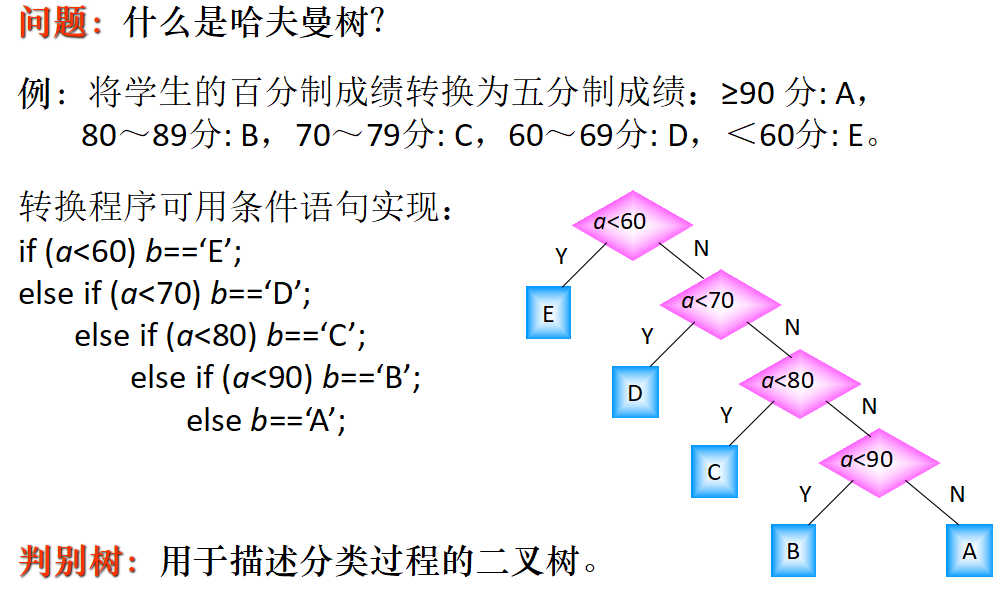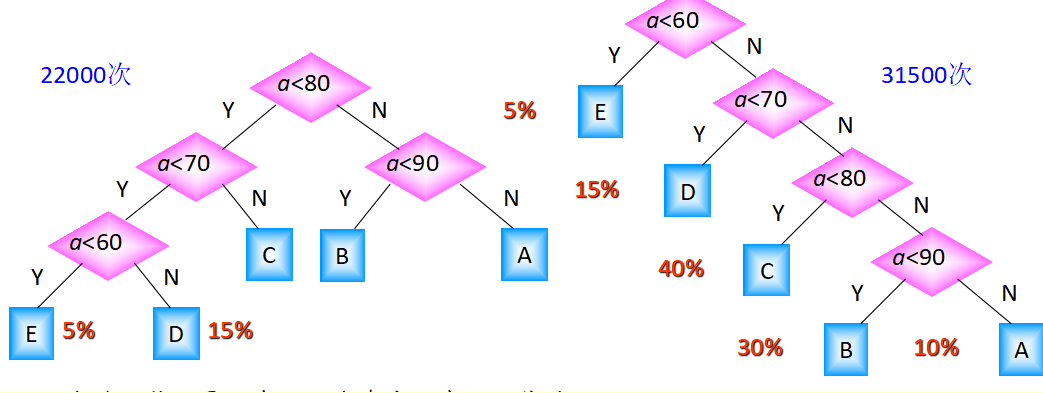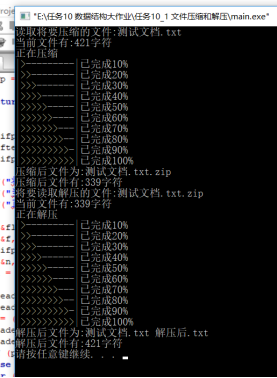• 利用哈夫曼编码进行对已有文件进行重新编码可以大大提高减小文件大小，减少存储空间。但是，这要求在首先对一个现有文件进行编码行成新的文件，也...在文件使用时，再对压缩文件进行解压缩，也就是译码，复原原有文件
• 写一个对文件进行压缩和解压缩的程序，功能如下： ① 可以对纯英文文档实现压缩和解压； ② 较好的界面程序运行的说明。 介绍哈夫曼： 效率最高的判别树即为哈夫曼树 在计算机数据处理中，霍夫曼编码...
写一个对文件进行压缩和解压缩的程序，功能如下：

① 可以对纯英文文档实现压缩和解压；

② 较好的界面程序运行的说明。

介绍哈夫曼：效率最高的判别树即为哈夫曼树

在计算机数据处理中，霍夫曼编码使用变长编码表对源符号（如文件中的一个字母）进行编码，其中变长编码表是通过一种评估来源符号出现机率的方法得到的，出现机率高的字母使用较短的编码，反之出现机率低的则使用较长的编码，这便使编码之后的字符串的平均长度、期望值降低，从而达到无损压缩数据的目的。

例如，在英文中，e的出现机率最高，而z的出现概率则最低。当利用霍夫曼编码对一篇英文进行压缩时，e极有可能用一个比特来表示，而z则可能花去25个比特（不是26）。用普通的表示方法时，每个英文字母均占用一个字节，即8个比特。二者相比，e使用了一般编码的1/8的长度，z则使用了3倍多。倘若我们能实现对于英文中各个字母出现概率的较准确的估算，就可以大幅度提高无损压缩的比例。

霍夫曼树又称最优二叉树，是一种带权路径长度最短的二叉树。所谓树的带权路径长度，就是树中所有的叶结点的权值乘上其到根结点的路径长度（若根结点为0层，叶结点到根结点的路径长度为叶结点的层数）。树的路径长度是从树根到每一结点的路径长度之和，记为WPL=（W1*L1+W2*L2+W3*L3+...+Wn*Ln），N个权值Wi（i=1,2,...n）构成一棵有N个叶结点的二叉树，相应的叶结点的路径长度为Li（i=1,2,...n）。可以证明霍夫曼树的WPL是最小的。

文件压缩与解压

姓名：  范天祚

1 程序说明

1.1数据结构

哈夫曼树

1.2函数功能说明

printfPercent界面

compress()读取文件内容并加以压缩，将压缩内容写入另一个文档

uncompress()解压缩文件，并将解压后的内容写入新文件

1.3 程序编写的思路及流程

压缩：统计字符出现次数、将节点按出现次数排序、构造哈夫曼树、设置字符编码、读文件字符、按设置好的编码替换字符、写入存储文件

解压：读取文件各参数、转换成二进制码、按码求对应字符、写入存储文件

#define _CRT_SECURE_NO_WARNINGS
#include <stdlib.h>
#include <stdio.h>
#include <string.h>

struct head
{
int b;						  //字符
long count;                   //文件中该字符出现的次数
long parent, lch, rch;        //make a tree
char bits;               //the huffuman code
};

struct head header, tmp;  //节点树

void printfPercent(int per)
{
int i = 0;
printf("|");
for(i = 0; i < 10; i++)
{
if(i < per/10)
printf(">");
else
printf("-");
}
printf("|已完成%d%%\n",per);
}

//函数：compress()
//作用：读取文件内容并加以压缩
//将压缩内容写入另一个文档
int compress(const char *filename,const char *outputfile)
{
char buf;
unsigned char c;
long i, j, m, n, f;
long min1, pt1, flength;
FILE *ifp, *ofp;
int per = 10;
ifp = fopen(filename, "rb");                  //打开原始文件
if (ifp == NULL)
{
printf("打开文件失败:%s\n",filename);
return 0;                             //如果打开失败，则输出错误信息
}
ofp = fopen(outputfile,"wb");                 //打开压缩后存储信息的文件
if (ofp == NULL)
{
printf("打开文件失败:%s\n",outputfile);
return 0;
}
flength = 0;
while (!feof(ifp))
{
fread(&c, 1, 1, ifp);
header[c].count ++;                       //读文件，统计字符出现次数
flength ++;                               //记录文件的字符总数
}
flength --;
header[c].count --;
for (i = 0; i < 512; i ++)                    //HUFFMAN算法中初始节点的设置
{
if (header[i].count != 0)
header[i].b = (unsigned char) i;
else
header[i].b = -1;
header[i].parent = -1;
header[i].lch = header[i].rch = -1;
}

for (i = 0; i < 256; i ++)                    //将节点按出现次数排序
{
for (j = i + 1; j < 256; j ++)
{
if (header[i].count < header[j].count)
{
tmp = header[i];
header[i] = header[j];
header[j] = tmp;
}
}
}

for (i = 0; i < 256; i ++)                    //统计不同字符的数量
{
if (header[i].count == 0)
break;
}

n = i;
m = 2 * n - 1;
for (i = n; i < m; i ++)
{
min1 = 999999999;
for (j = 0; j < i; j ++)
{
if (header[j].parent != -1) continue;
if (min1 > header[j].count)
{
pt1 = j;
min1 = header[j].count;
continue;
}
}
header[i].count = header[pt1].count;
header[pt1].parent = i;
header[i].lch = pt1;
min1 = 999999999;
for (j = 0; j < i; j ++)
{
if (header[j].parent != -1) continue;
if (min1 > header[j].count)
{
pt1 = j;
min1 = header[j].count;
continue;
}
}
header[i].count += header[pt1].count;
header[i].rch = pt1;
header[pt1].parent = i;
}

for (i = 0; i < n; i ++)                        //构造HUFFMAN树，设置字符的编码
{
f = i;
header[i].bits = 0;
while (header[f].parent != -1)
{
j = f;
f = header[f].parent;
if (header[f].lch == j)
{
j = strlen(header[i].bits);
memmove(header[i].bits + 1, header[i].bits, j + 1);
header[i].bits = '0';
}
else
{
j = strlen(header[i].bits);
memmove(header[i].bits + 1, header[i].bits, j + 1);
header[i].bits = '1';
}
}
}

//下面的就是读原文件的每一个字符，按照设置好的编码替换文件中的字符
fseek(ifp, 0, SEEK_SET);                                                //将指针定在文件起始位置
fseek(ofp, 8, SEEK_SET);                                //以8位二进制数为单位进行读取
buf = 0;
f = 0;
pt1 = 8;

printf("读取将要压缩的文件:%s\n",filename);
printf("当前文件有:%d字符\n",flength);
printf("正在压缩\n");

while (!feof(ifp))
{
c = fgetc(ifp);
f ++;
for (i = 0; i < n; i ++)
{
if (c == header[i].b) break;
}
strcat(buf, header[i].bits);
j = strlen(buf);
c = 0;
while (j >= 8)                                             //当剩余字符数量不小于8个时
{
for (i = 0; i < 8; i ++)                               //按照八位二进制数转化成十进制ASCII码写入文件一次进行压缩
{
if (buf[i] == '1') c = (c << 1) | 1;
else c = c << 1;
}
fwrite(&c, 1, 1, ofp);
pt1 ++;
strcpy(buf, buf + 8);
j = strlen(buf);
}
if(100 * f/flength > per)
{
printfPercent(per);
per += 10;
}
if (f == flength)
break;
}
printfPercent(100);

if (j > 0)                                                      //当剩余字符数量少于8个时
{
strcat(buf, "00000000");
for (i = 0; i < 8; i ++)
{
if (buf[i] == '1') c = (c << 1) | 1;
else c = c << 1;                                        //对不足的位数进行补零
}
fwrite(&c, 1, 1, ofp);
pt1 ++;
}
fseek(ofp, 0, SEEK_SET);                                        //将编码信息写入存储文件
fwrite(&flength,1,sizeof(flength),ofp);
fwrite(&pt1, sizeof(long), 1, ofp);
fseek(ofp, pt1, SEEK_SET);
fwrite(&n, sizeof(long), 1, ofp);
for (i = 0; i < n; i ++)
{
tmp = header[i];

fwrite(&(header[i].b), 1, 1, ofp);
pt1++;
c = strlen(header[i].bits);
fwrite(&c, 1, 1, ofp);
pt1++;
j = strlen(header[i].bits);

if (j % 8 != 0)                                             //当位数不满8时，对该数进行补零操作
{
for (f = j % 8; f < 8; f ++)
strcat(header[i].bits, "0");
}

while (header[i].bits != 0)
{
c = 0;
for (j = 0; j < 8; j ++)
{
if (header[i].bits[j] == '1') c = (c << 1) | 1;
else c = c << 1;
}
strcpy(header[i].bits, header[i].bits + 8);
fwrite(&c, 1, 1, ofp);                                            //将所得的编码信息写入文件
pt1++;
}

header[i] = tmp;
}
fclose(ifp);
fclose(ofp);                                                              //关闭文件

printf("压缩后文件为:%s\n",outputfile);
printf("压缩后文件有:%d字符\n",pt1 + 4);

return 1;                                       //返回压缩成功信息
}

//函数：uncompress()
//作用：解压缩文件，并将解压后的内容写入新文件
int uncompress(const char *filename,const char *outputfile)
{
char buf, bx;
unsigned char c;
char out_filename;
long i, j, m, n, f, p, l;
long flength;
int per = 10;
int len = 0;
FILE *ifp, *ofp;
char c_name = {0};
ifp = fopen(filename, "rb");                                              //打开文件
if (ifp == NULL)
{
return 0;     //若打开失败，则输出错误信息
}

//读取原文件长
if(outputfile)
strcpy(out_filename,outputfile);
else
strcpy(out_filename,c_name);

ofp = fopen(out_filename, "wb");                                            //打开文件
if (ofp == NULL)
{
return 0;
}

fseek(ifp,0,SEEK_END);
len = ftell(ifp);
fseek(ifp,0,SEEK_SET);

printf("将要读取解压的文件:%s\n",filename);
printf("当前文件有:%d字符\n",len);
printf("正在解压\n");

fread(&flength, sizeof(long), 1, ifp);                                    //读取原文件长
fread(&f, sizeof(long), 1, ifp);
fseek(ifp, f, SEEK_SET);
fread(&n, sizeof(long), 1, ifp);                                          //读取原文件各参数
for (i = 0; i < n; i ++)                                                  //读取压缩文件内容并转换成二进制码
{
fread(&header[i].b, 1, 1, ifp);
fread(&c, 1, 1, ifp);
p = (long) c;
header[i].count = p;
header[i].bits = 0;
if (p % 8 > 0) m = p / 8 + 1;
else m = p / 8;
for (j = 0; j < m; j ++)
{
fread(&c, 1 , 1 , ifp);
f = c;
_itoa(f, buf, 2);
f = strlen(buf);
for (l = 8; l > f; l --)
{
strcat(header[i].bits, "0");                                  //位数不足，执行补零操作
}
strcat(header[i].bits, buf);
}
header[i].bits[p] = 0;
}

for (i = 0; i < n; i ++)
{
for (j = i + 1; j < n; j ++)
{
if (strlen(header[i].bits) > strlen(header[j].bits))
{
tmp = header[i];
header[i] = header[j];
header[j] = tmp;
}
}
}

p = strlen(header[n-1].bits);
fseek(ifp, 8, SEEK_SET);
m = 0;
bx = 0;

while (1)
{
while (strlen(bx) < (unsigned int)p)
{
fread(&c, 1, 1, ifp);
f = c;
_itoa(f, buf, 2);
f = strlen(buf);
for (l = 8; l > f; l --)
{
strcat(bx, "0");
}
strcat(bx, buf);
}
for (i = 0; i < n; i ++)
{
if (memcmp(header[i].bits, bx, header[i].count) == 0) break;
}
strcpy(bx, bx + header[i].count);
c = header[i].b;
fwrite(&c, 1, 1, ofp);
m ++;

if(100 *  m/flength > per)
{
printfPercent(per);
per += 10;
}
if (m == flength) break;
}
printfPercent(100);

fclose(ifp);
fclose(ofp);

printf("解压后文件为:%s\n",out_filename);
printf("解压后文件有:%d字符\n",flength);

return 1;                   //输出成功信息
}

int main(int argc,const char *argv[])
{
memset(&header,0,sizeof(header));
memset(&tmp,0,sizeof(tmp));

compress("测试文档.txt","测试文档.txt.zip");
uncompress("测试文档.txt.zip","测试文档.txt 解压后.txt");
system("pause");

return 0;
}


2 功能展示

2.1 控制台显示2.2 文件效果

开始时只有一个文件《测试文档.txt》：打开《测试文档.txt》《测试文档.txt》文件大小：程序运行结束后多了两个文件：以文本形式打开压缩二进制文件《测试文档.txt.zip》：《测试文档.txt.zip》文件属性：展开全文• C语言的学习资料，李丽娟的学习视频很使用C的初学者，我就是从此学起的！
• c语言实现文件压缩，用huffman编码实现。并修改注册表实现鼠标右击出现如同rar的简单操作。
• 基于Huffman编码的C语言压缩和解压缩文件程序文件程序。 c功底比较差。。。一下午只写出了huffman树。 想求能直接调试的代码。最好有注释。c 编码
• 主要介绍了C语言压缩文件和用MD5算法校验文件完整性的实例教程,这里演示了Windows下将文件压缩为7z格式以及MD5检验文件和密码的方法,需要的朋友可以参考下
• 将编码表写入默认文件当中,并在结尾存入叶子节点数（count）与压缩文件的总bit数 1111000 27 ........... ........... #sum_bit##count# */ void freToFile(int code[],HCode *HC) { int i; //打开默认...
#include<stdio.h>
#include<stdlib.h>
#include<string.h>
#include<math.h>
//极大值用于生成Huffman树
#define MAXSIZE 100000000
//用于生成相应叶子节点Huffman编码的二维字符数组
typedef char* HCode;
//Huffman树节点
typedef struct node
{
int weight;
int data;
int parent,lchild,rchild;
}Node;
//count 叶子节点数的计算  sum_bit 记录被压缩文件编码后的编码总长度
int sum_bit,count;
//Huffman叶子节点，最多不过256个（以字节为单位）
Node huffmanNode;
//解压缩的时候记录每次读取到编码（0....1..）
int num;
//对应词频信息表的信息，用ASCII值表示
int code;
//二维字符数组
HCode *HC;
//统计词频时用于查找是否已经记录过,记录过的话返回下标，没有则返回0
int isInNode(int value)
{
int i = 1;
for(;i<=count;i++)
{
if(huffmanNode[i].data == value)
{
return i;
}
}
return 0;
}
//获取文件词频，记录在Node huffmanNode的节点数组当中
void  calWeight(char *file)
{
count = 0;
FILE *f;
int a;
//以二进制方式打开文件，为了读取到换行符
f = fopen(file,"rb");
if(f == NULL)
{
printf("文件不存在！打开失败！");
return ;
}
while(!feof(f))
{
a = fgetc(f);
if(a==EOF) break;
if(!isInNode(a))   //count从1开始计数
{
count++;
huffmanNode[count].weight = 1;
huffmanNode[count].data   = a;
}
else
{
int i = isInNode(a);
huffmanNode[i].weight++;
}
}
fclose(f);
}

/*得到待压缩文件的总字节数，权值为几就代表着有多少个字节*/
int getSumBytes()
{
int i=1;
int result = 0;
for(;i<=count;i++)
{
result +=huffmanNode[i].weight;
}
return result;
}

//获取压缩后文件的总bit数
int getSumBits()
{
int i = 1;
int result = 0;
for(;i<=count;i++)
{
result+=huffmanNode[i].weight * strlen(HC[i]);
}
return result;
}

//建立huffman树 根据huffman树的特性,具有n个节点的huffman树的具有2n-1个节点
//n值由全局变量count值来确定，该函数主要用来初始化Huffman树的所有节点信息
void  createHufmanTree(Node * huffmanTree)
{
int m = 2*count - 1;
int m1,m2,x1,x2,i,j;
//初始化结点信息，从1--count这count个节点信息为叶子节点的信息
for(i=1;i<=count;i++)
{

huffmanTree[i].data = huffmanNode[i].data;
huffmanTree[i].lchild = -1;
huffmanTree[i].rchild = -1;
huffmanTree[i].parent = -1;
huffmanTree[i].weight = huffmanNode[i].weight;
}
//从count---2*count-1这些节点首先初始化为空
for(;i<=m;i++)
{
huffmanTree[i].data = 0;    huffmanTree[i].weight = 0;
huffmanTree[i].lchild = -1; huffmanTree[i].rchild = -1;
huffmanTree[i].parent = -1;
}
//构造huffman树，按照huffman树构建原理
for(i=count+1;i<=m;i++)
{
/*m2,m1分别存储倒数第二小的权值和倒数第一小的权值
x2,x1分别存储倒数第二小的下标和倒数第一小的下标*/
m1 = m2 = MAXSIZE;
x1 = x2 = 0;
for(j=1;j<i;j++)
{
if(huffmanTree[j].parent == -1&&huffmanTree[j].weight <m1)
{
m2 = m1;                    x2 = x1;
m1 = huffmanTree[j].weight; x1 = j;
}
else if(huffmanTree[j].parent == -1&&huffmanTree[j].weight<m2)
{
m2 = huffmanTree[j].weight;
x2 = j;
}

}
/*合并成一颗新的树*/
huffmanTree[x1].parent = i; huffmanTree[x2].parent = i;
huffmanTree[i].lchild = x1; huffmanTree[i].rchild = x2;
huffmanTree[i].weight = m1+m2;
}
}

/*字符编码，从构建好的Huffman树当中读取每个叶子节点的huffman编码，并将叶子节点的信息放入到code[]中*/
HCode * getHuffmanCode(Node * huffmanTree,HCode *HC,int code[])
{
int i = 1,c,start,f;
//构造了字符编码的字符数组共有count+1个 通过读取一个复制一个的方式完成编码获取

char * cd = (char *)malloc((count+1)*sizeof(char));
//还是这个问题的
cd[count] = '\0';
for(;i<=count;i++)
{
start = count;
for(c=i,f=huffmanTree[i].parent;f!=-1;c=f,f=huffmanTree[f].parent)
{
if(huffmanTree[f].lchild == c)  cd[--start] = '0';
else cd[--start] = '1';
}
//为每个字符数组分配相应的数量 由于范围的问题要仔细考虑的
HC[i] = (char *)malloc((count+1-start)*sizeof(char));
//参数均为char *
strcpy(HC[i],&cd[start]);
code[i] = huffmanTree[i].data;
}
return HC;
}
/*
将编码表写入默认文件当中,并在结尾存入叶子节点数（count）与压缩后文件的总bit数
1111000  27
...........
...........
#sum_bit##count#
*/
void freToFile(int code[],HCode *HC)
{
int i;
//打开默认文件
FILE *fe = fopen("C:\\dic.txt","wb");
//将编码信息和叶子节点信息写入词典
for(i=1;i<=count;i++)
{
fprintf(fe,"%s %d\n",HC[i],code[i]);
}
char c = '#';
//写入sum_bit
fprintf(fe,"%c",c);
fprintf(fe,"%d",getSumBits());
fprintf(fe,"%c",c);
//写入count
fprintf(fe,"%c",c);
fprintf(fe,"%d",count);
fprintf(fe,"%c",c);

fclose(fe);
}
//由于词频表是按照字符串方式存储的叶子节点信息,读取出来的字符串需要转换成int值再使用
//其中需要使用pow函数，由于pow函数有精度损失，自己写了一个使用
int powmy(int a,int b)
{
if(b==0) return 1;
int i = 0;
int result = 1;
for(;i<b;i++)
{
result *=a;
}
return result;
}

/*从编码表文件读取相应信息以用来解压文件，读取信息包括编码和叶子信息*/
HCode* freFromFile(int code[],HCode *HC)
{
int i;
FILE *fe = fopen("C:\\dic.txt","rb");
if(fe==NULL)
{
printf("词典文件不存在！");
return NULL;
}
int k;
int num;
int m;
int flag = 0;
char * cd = (char *)malloc((256+1)*sizeof(char));
//读取一个字节
char c = fgetc(fe);
for(i=1;flag!=1;i++)
{
//如果读取到#号键，就跳出循环，继续读取sum_bit和count值
if(c=='#') break;
//每一行的读取直到读到空格，然后就完成了一条huffman编码的读取
int j = 0;
while(c!=' ')
{
cd[j++] = c;
c = fgetc(fe);
}
cd[j] = '\0';

//将读取到的huffman编码存入相应的二维字符数组当中去
HC[i] = (char *)malloc((j+1)*sizeof(char));
strcpy(HC[i],&cd);
//下面直到读取到空格键为止，读取huffman叶子节点信息，读取到的是字符，需要转换成int值
c = fgetc(fe);

k = 0;
while(c!='\n')
{
num[k++] = c-'0';
c = fgetc(fe);
}
code[i] = 0;
m = 0;
//转换成int值，存入code[]数组当中
for(k=k-1;k>=0;k--)
{
code[i]+=num[k]*powmy(10,m);
m++;
}
//继续向下读取
c = fgetc(fe);
}
//获取压缩文件的总bit数，以用来判断最后一次读取的是不是足够8位
c = fgetc(fe);
k = 0;
while(c!='#')
{
num[k++] = c-'0';
c = fgetc(fe);
}
//同样将读取到的char转换成int
m = 0;
sum_bit = 0;
for(k=k-1;k>=0;k--)
{
sum_bit+=(num[k]*powmy(10,m));
m = m + 1;
}

c = fgetc(fe);  c = fgetc(fe);//头一个读取#，后一个才开始读取数据
k = 0;
while(c!='#')
{
num[k++] = c-'0';
c = fgetc(fe);
}
//将读取到的char转换成int
m = 0;  count = 0;
for(k=k-1;k>=0;k--)
{
count+=num[k]*pow(10,m);
m++;
}
fclose(fe);
return HC;
}

/*压缩文件*/
void compress_file(char* file1,char*file2)
{
int i,sum = 0,flag = 0,j,k = 0;
//数组开设的不够大是最后的一个bug的成因，因为有可能这个Huffman编码很长很长
int eight;
memset(eight,0,1000*sizeof(int));
FILE *fo = fopen(file1,"rb");
FILE *fw = fopen(file2,"wb");
if(fo == NULL||fw == NULL)
{
printf("文件读取失败！");
return;
}
//统计已经压缩的字节总数，用于计算压缩百分比
int aa = 0;
int sum_bytes = getSumBytes();
while(!feof(fo))
{
sum = 0;
int a = fgetc(fo);
//每次读取一个字节就+1
aa++;
//读取了一个字节之后就与编码表进行比较，查找对应的编码
for(i=1;i<=count;i++)
{
if(code[i] == a)
{
//flag作为计数器，当凑够8位之后就作为一个字节写入压缩文件
flag+=strlen(HC[i]);
int len = strlen(HC[i]);
//flag 小于8的时候继续累加，直到凑够8个
if(flag<8)
{
for(j=0;j<len;j++)
eight[k++] = HC[i][j]-'0';/*我们存储的是字符串，是多少就是多少*/
}
//当flag>=8的时候，将8位写进压缩文件，同时将剩余的没有写入的huffman编码重新移到
//eight【】数组前面去，同时修改flag
else if(flag>=8)
{
//将匹配到的huffman编码写进8位数组，直到k值为8，k值始终代表现在eight【】数组的长度
for(j=0;k<8;j++)
eight[k++] = HC[i][j]-'0';
//将匹配到的huffman编码的没有完全写进去的添加到后面。
for(;j<len;j++)
eight[k++] = HC[i][j]-'0';
//计算8位对应的int值，写入文件
sum+=eight*128+eight*64+eight*32+eight*16+eight*8
+eight*4+eight*2+eight*1;
//前8为置0
for(j=0;j<8;j++)
eight[j] = 0;
//将后面的移植到前面去
for(j=8;j<k;j++)
eight[j-8] = eight[j];
//重置flag与k
k = flag = j-8;
//写进文件
char c = sum;
fputc(c,fw);

if(aa%1000==0)
{
printf("\r正在进行压缩，请稍等……%6.2f%%",(double)aa/sum_bytes*100.0);
}
fflush(fw);
i = count+1;
}
}
}
}
aa = sum_bytes;
printf("\r正在进行压缩，请稍等……%6.2f%%",(double)aa/sum_bytes*100.0);
printf("压缩成功！");
/*考虑到最后可能没有凑够八位的情况*/
if(flag)
{
sum+=eight*128+eight*64+eight*32+eight*16+eight*8
+eight*4+eight*2+eight*1;
char c = sum;
fputc(c,fw);
sum_bit +=flag;
fflush(fw);
}
fclose(fw);
fclose(fo);
}

/*用于在解压的时候将读取到的ASCII码转换为二进制数*/
int  swap(int data)
{
int i = 0;
while(data)
{
num[i++] = data%2;
data = data/2;
}
return i;
}

/*进行文件的解压*/
void uncompress_file(char* file1,char* file2)
{

FILE *fo = fopen(file1,"rb");
FILE *fw = fopen(file2,"wb");
if(fo==NULL ||fw == NULL)
{
printf("文件打开失败！");
return;
}
char str;
int i,j,k,temp = 0;
int index;
int sum_bit2 = sum_bit;
//直到读取到文件结尾
while(!feof(fo))
{
if(sum_bit2<0) break;
//读取一次，减去8位
sum_bit2 -=8;
int data = fgetc(fo);
if(data == -1) break;
//index用来在sum_bit2小于0的时候设置读取为位数（也就是说最后不用读取8位了）
if(sum_bit2<0)
{
index = 0-sum_bit2;
}
else
{
index = 0;
}
if(data == -1) break;
memset(num,0,sizeof(num));
//将读取到的data转换成二进制数
swap(data);
i = temp;
//将转换后的二进制数变为字符串，注意顺序
//是一位一位的往里面填，填进去一位立即进行比较，当找到相应的信息就调出来
for(k=7;k>=index;i++,k--)
{
if(num[k])
str[i] = '1';
else
str[i] = '0';

str[i+1] ='\0';
//查找编码表当中与该字符串（编码）相同的信息，然后将叶子信息写入解压文件
for(j=1;j<=count;j++)
{
if(strcmp(str,HC[j])==0)
{
//将叶子信息写入到文件（写入的是int值，是该int值表示的字符）
fputc(code[j],fw);
if((sum_bit-sum_bit2)%1500==0)
{
printf("\r文件正在解压中,请耐心等待……%6.2f%%",(double)(sum_bit-sum_bit2)/sum_bit*100.0);
}

fflush(fw);
j = count+1;
i = -1;
}
}
}
if(i)
{
temp = i;
}
else
{
temp = 0;
}
}
sum_bit2 = 0;
printf("\r文件正在解压中,请耐心等待……%6.2f%%",(double)(sum_bit-sum_bit2)/sum_bit*100.0);
printf("解压成功！");
fclose(fw);
fclose(fo);
}

int main(int argc, char **argv)
{
if(strcmp(argv,"-c")==0)
{
//获取文件的词频
calWeight(argv);
//申请Huffman树的内存，已经获得叶子节点数，根据节点总数与叶子节点数的关系分配内存
Node *huffmanTree = (Node *)malloc((2*count-1+1)*sizeof(Node));
//创建Huffman树
createHufmanTree(huffmanTree);
//为Huffman编码表申请一个二维的字符数组指针
HC = (HCode *)malloc((count+1)*sizeof(HCode));
//向指针赋值，getHuffmanCode()函数返回编码表
HC = getHuffmanCode(huffmanTree,HC,code);
//根据编码表HC和编码对应的data表code压缩文件
compress_file(argv,argv);
//将编码存入到默认的编码表当中（C:\\dic.txt）
freToFile(code,HC);
}
else if(strcmp(argv,"-u")==0)
{
//为编码表分配内存，由于不知道叶子节点数，分配257
HC = (HCode *)malloc(257*sizeof(HCode));
//从词频表当中获取编码
HC = freFromFile(code,HC);
//根据编码表和data表解压文件
uncompress_file(argv,argv);
}
return 0;
}


展开全文• 哈夫曼编码是一种常用的数据压缩技术，对数据文件进行哈夫曼编码可大大缩短文件的传输长度，提高信道利用率及传输效率。要求采用哈夫曼编码原理，统计文本文件中字符出现的词频，以词频作为权值，对文件进行哈夫曼...
• c语言文件的写法和字符的压缩，有关于文件的书模样，这个跟ＩＴＡＴ大赛的的题目有关，有兴趣的可以下载来看看，忘大家支持一下。给一些积分下个软件
• 这是用c语言写的 采用huffman算法做的数据结构的课程设计。
• 用优先队列构造huffman树，然后压缩编码，由8个字符串的huffman编码转换成unsinged char,保存到压缩文件，从而实现压缩. 要对文件进行解压缩，要将编码的huffman树保存到 压缩文件，否则就没有解码信息了. 要在...
• C语言】【数据结构】【压缩、解压缩】利用哈夫曼编码进行对已有文件进行重新编码可以大大提高减小文件大小，减少存储空间。这要求在首先对一个现有文件进行编码行成新的文件，也就是压缩。在文件使用时，再对压缩...
• 符号表结构体： struct node { // 字符串形式存储的Huffman编码 ... // 这个字符在文件中出现的次数 long count; // 在生成Huffman树的时候是否已经被当作叶子节点 int checked; // 符号 ...


符号表结构体：

struct node
{
// 字符串形式存储的Huffman编码
char code[MAX_CODE_LENGTH];
// 这个字符在文件中出现的次数
long count;
// 在生成Huffman树的时候是否已经被当作叶子节点
int checked;
// 符号
char sym;
// left和right只在生成Huffman树的时候有用
struct node* next,*left,*right;
};

全局变量：

const int BIT_WIDTH_CHAR = 8;
const int END_SYM_FLAG = 200;// 符号的范围是-127~128
int gl_total_node_num = 0;// 符号表的总长度
int gl_source_file_length = 0;// 源文件的总长度

辅助函数：

// 在链表中查找指定的字符
// 参数：符号表
// 参数：字符
// 返回值：找到的节点的指针
struct node* content_char(struct node*,char);
// 在链表中查找指定编码
// 参数：符号表
// 参数：编码
// 返回值：找到的节点的指针
struct node* content_code(struct node* list,const char* ch);
// 根据字符创建一个新的节点
// 参数：字符
// 参数：计数
// 返回值：新节点指针
struct node* create_new_node(char ch,int count);
// 插入新节点到符号表的最前面
// 参数list：目标链表
// 参数new_node：新节点
// 返回值：插入后的链表
struct node* insert_node(struct node *list,struct node *new_node);
// 输出链表
// 参数list：目标链表
void print_list(struct node *list);
// 获取到最小的未被检查的count的节点，返回它的指针，并将其设置为检查过的
// 参数list_addr：链表头
// 返回值：第一个未检查的最少出现次数的结点指针
struct node* get_smallest_node(struct node *list_addr);

压缩：

第一步：建立符号表

在main函数中：获取文件的总长度，用于生成进度条

// 获取文件长度，以实现进度条
fseek(source_file,0,SEEK_END);
gl_source_file_length = ftell(source_file);
fseek(source_file,0,SEEK_SET);


扫描源文件，按字符读取，建立符号表，统计每个字符出现的次数

首先提示进度条，10个'.'为结束

将读取到的字符在已有的符号表中查找，如果已存在就将该节点的count+1，否则就创建新节点并插入到符号表中

统计符号表中总节点数

// 生成符号链表（含频率）
// 参数：源文件
// 参数：目标链表
// 返回值：符号链表
struct node* generate_count(FILE* f,struct node*list)
{
printf("counting");
int count=0;

char ch;
struct node *content_node;
while(fread(&ch,sizeof(char),1,f)==1)
{
// 进度条
count++;
if(count%(gl_source_file_length/10+1)==0)
printf(".");

// 插入符号表
content_node = content_char(list,ch);
if(content_node)
content_node->count++;
else
{
gl_total_node_num++;
list=insert_node(list,create_new_node(ch,1));
}
}
printf("\n");
return list;
}

第二步：生成Huffman树

生成Huffman树

使用先前统计的符号表总数进行计数循环，因为生成树的所有非叶子节点共有叶子节点-1个

首先输出进度条

视初始时所有的符号表中的节点为只有一个节点的子树，获取两个最小的count的子树根节点指针，这是通过节点的checked属性实现的，如果使用了两个子树根节点生成新的子树，那么这两个子树根节点的checked将会设为1，在寻找最小节点的时候将会跳过

将新的树的中间节点插入到符号表中

最终的效果是符号表中只有最前面的一个节点的checked为0

// 生成Huffman树
// 参数：符号链表
// 返回值：含有Huffman树的链表，非叶节点将插入到链表前面，并有左右孩子属性，叶节点没有左右孩子属性
struct node* generate_tree(struct node* list)
{
printf("generate_tree");
// 生成树
for(int i=1;i<gl_total_node_num;i++)
{
// 进度条
if(i%(gl_total_node_num/10+1)==0)
printf(".");

// 获取最小出现次数的两个符号，并生成新的节点插入符号表
struct node *sm_left=get_smallest_node(list);
struct node *sm_right=get_smallest_node(list);
struct node *new_node=create_new_node('\0',sm_left->count+sm_right->count);
new_node->left=sm_left;
new_node->right=sm_right;

list = insert_node(list,new_node);
}
printf("\n");
return list;
}

第三步：生成编码

从Huffman树中生成符号表的编码属性

因为这是一棵树，所以用递归的方式进行

某节点的左孩子的编码将在继承其父节点编码的基础上扩展'0'，右孩子将在继承其父节点的编码的基础上扩展'1'

最终所有叶子节点的编码就是Huffman编码

// 递归的生成Huffman编码于符号链表
// 参数：Huffman树
void generate_code(struct node*list)
{
// 生成Huffman编码
if(!list)return;
// 左子树扩展'0'
if(list->left)
{
strcat(list->left->code,list->code);
strcat(list->left->code,"0");
generate_code(list->left);
}
// 右子树'1'
if(list->right)
{
strcat(list->right->code,list->code);
strcat(list->right->code,"1");
generate_code(list->right);
}
}

因为之后树就没什么用了，只有叶子节点有用，所以将其他节点释放掉

// 释放Huffman树的非叶子节点，只留下符号链表
// 参数：Huffman树
// 返回值：不含Huffman树的符号链表
struct node* free_tree(struct node*list)
{
struct node*free_node=list;
while(list->left && list->right)// 左右子树不为空的节点都是需要释放的
{
free_node=list;
list=list->next;
free(free_node);
}
return list;
}

第四步：生成目标文件

首先将符号表写入到目标文件头部，再次扫描源文件，将每个字符转换为对应的编码，并以二进制的形式存储在目标文件中

// 生成目标文件
// 参数：源文件
// 参数：目标文件
// 参数：符号链表
void generate_des_file(FILE* sf,FILE* df,struct node*list)

首先，为了解压，要把符号表的内容写入到目标文件前面

其中符号和编码时两两配对的，最后通过一个符号表不可能取到的值标记结束

标识符之间是通过空格隔开，最终结束时以换行符结尾。这里的规则将在解压的时候需要严格遵守

// 符号表以文本形式写入到目标文件的前端，解压时需要的信息
struct node* index=list;
while(index)
{
fprintf(df,"%d %s ",index->sym,index->code);
index=index->next;
}
// 指示结尾，"END"实际上没有用到，只用于和END_SYM_FLAG配对
fprintf(df,"%d %s\n",END_SYM_FLAG,"END");


变量：

// 实际文件内容（二进制形式）
// 前者是从源文件中读取的字符，后置是对Huffman编码进行二进制形式转换后取8位形成的字符
char ch,des_ch='\0';
// 目标字符知否足够8位可以进行写入？
int des_ch_length=0;
// 因为之前进行了读取，所以这里回到文件头
fseek(sf,0,SEEK_SET);
int count=0;// 程序执行进度提示


while循环读文件：

while(fread(&ch,sizeof(char),1,sf)==1)

输出进度条，并根据从源文件中读取的字符找符号表中对应的节点

// 进度条
count++;
if(count%(gl_source_file_length/10+1)==0)
printf(".");

// 在符号表中找这个字符
// 没有找到一定是出错了
struct node *content_node = content_char(list,ch);
if(!content_node)
{
printf("error:cannot match with sym list\n");
exit(0);
}


现在需要将符号对应的字符串Huffman编码转化为一个个8位char类型，其中每一位代表一位Huffman编码

对这个编码每一位循环处理：将已经部分生成的目标字符左移一位，如果当前的编码位为1就用掩码0000 0001和目标字符按位或，那么最后一位将为1，其余不变，当前编码位为0就不做操作，因为本来左移就补0

当记录的长度达到8的时候就将这个字符写入到目标文件，将记录长度清零，继续对编码的每一位循环

// 对这个符号对应的Huffman编码进行二进制转化
char* current_code=content_node->code;
for(int i=0;i<strlen(current_code);i++)
{
// 每次循环左移一位，长度+1
des_ch=des_ch<<1;
des_ch_length++;
// 末位默认位0，否则置1
if(current_code[i]=='1')des_ch |= (char)1;

// 已经足够了一个字符，就写入，并清0
if(des_ch_length==8)
{
if(!fwrite(&des_ch,sizeof(char),1,df))
{
printf("error:cannot write to des file.\n");
exit(0);
}
des_ch_length=0;
des_ch=0;
}// 形成了一个字符
}// Huffman编码


但是最后一位不一定够8位，这需要额外的说明

将剩下的这个字符左对齐，并在目标文件的最后一个字符说明前一个字符有几位有效

// 最后一个字符，没有满足8位
if(des_ch_length!=0)
{
des_ch=des_ch<<BIT_WIDTH_CHAR-des_ch_length;
if(!fwrite(&des_ch,sizeof(char),1,df))
{
printf("error:cannot write to des file.\n");
exit(0);
}
}
// 最后这个一定是一个字符（1-8），表示最后一个有效字符的长度
fprintf(df,"%d",des_ch_length);


解压：

第一步：从目标文件读取符号表

首先从源文件头读取并创建符号表

值得注意的是读取字符串的时候用fscanf将会在遇到空格的时候结束，而用其他的方式将会在换行符时结束，但是我们在生成的时候全都生成在一行

在读取结束的时候需要将换行符读掉，否则接下来将会读到换行符并解析

// 获取文件头的符号表
// 参数：源文件
// 返回值：符号表
struct node* de_get_sym_list(FILE* f)
{
printf("getting sym list...\n");

char code[MAX_CODE_LENGTH];
int ch_int;
struct node* list=NULL;

fscanf(f,"%d %s",&ch_int,code);
// 如果没有读到结束标志
while(ch_int!=END_SYM_FLAG)
{
// 创建新节点并插入符号表
struct node* new_node=create_new_node((char)ch_int,0);
strcpy(new_node->code,code);
list=insert_node(list,new_node);
fscanf(f,"%d %s",&ch_int,code);
}
fgetc(f);// 将换行符读掉，按照生成的规则，最后是一个换行符
return list;
}

第二步：解析压缩内容

// 生成解压后的文件
// 参数：源文件
// 参数：目标文件
// 参数：符号链表
void de_generate_des_file(FILE* sf,FILE* df,struct node* list)

变量：

// 存储未形成一个有效的Huffman编码的字符串
char temp_code[MAX_CODE_LENGTH] = {'\0'};
int last_length;// 最后一个字符的有效位长度
// 分别指向实际的压缩内容（去除头部的符号表）的头和尾
long current_file_index,file_length;
// 位操作的掩码，首位为1其余为0
char mask=((char)1)<<BIT_WIDTH_CHAR-1;
// 用于扩展Huffman编码的字符串，"0"或者"1"
char append[]={'0','\0'};


对文件的长度进行测量，并将最后一个字符有效位数读取进来，如果不记录长度，在之后的循环读取中想要跳过最后一个符号将会有些麻烦

记得要将文件的游标移动到合适的位置

// 记录此时符号表读取结束的位置
current_file_index = ftell(sf);
// 从文件尾获取最后一个字符的长度，以及文件的长度
fseek(sf,-(sizeof(char)),SEEK_END);
file_length = ftell(sf);
last_length = fgetc(sf)-'0';
// 回复当前位置到符号表结束的位置
fseek(sf,current_file_index,SEEK_SET);


因为已知了长度，所以读文件的时候可以计数循环，而不是检测文件尾。跳过最后一个字符（当然，这里的最后一个不包括后面的那个表示它的有效位数的数字字符）

for(int i=current_file_index;i<file_length-1;i++)

首先时进度条，并进行读取：

// 进度条
if(i%((file_length-current_file_index)/10+1)==0)
printf(".");
// 读取是否成功
if(fread(&ch,sizeof(char),1,sf)!=1)
{
if(ferror(sf))
printf("error:cannot read file.\n");
if(feof(sf))
printf("end of reading file.\n");
exit(0);
}


对这个字符的每一位循环，将这个位转化为字符形式扩展到已有的字符串上，每扩展一位就检测一下符号表中有没有这个编码，有了就将对应的字符输出到文件中，并将字符串清空

位操作需要获取的是第一个位，所以掩码是第一位为1其余为0，按位与将获取第一个位：非0为1，0为0

// 将这一个字符的每一位扩展到未竟的Huffman编码上
for(int j=0;j<BIT_WIDTH_CHAR;j++)
{
append='0' + ((ch & mask)==0?0:1);
strcat(temp_code,append);
ch=ch<<1;
// 尝试在符号表中寻找
the_node = content_code(list,temp_code);
if(the_node)
{
// 如果找到了就把其代表的符号写入文件
if(fwrite(&(the_node->sym),sizeof(char),1,df)!=1)
{
printf("error:failed to write file.\n");
exit(0);
}
// 清零临时字符串
temp_code='\0';
}// 如果在符号表中找到
}// 对一个字符的每一位


最后一个字符，利用之前的长度进行循环，而不是8位

// 最后一个字符
if(fread(&ch,sizeof(char),1,sf)!=1)
{
printf("error:cannot read file.\n");
exit(0);
}
for(int i=0;i<last_length;i++)
{
append='0' + ((ch & mask)==0?0:1);
strcat(temp_code,append);
ch=ch<<1;
// 尝试在符号表中寻找
the_node = content_code(list,temp_code);
if(the_node)
{
// 如果找到了就把其代表的符号写入文件
if(fwrite(&(the_node->sym),sizeof(char),1,df)!=1)
{
printf("error:failed to write file.\n");
exit(0);
}
// 清零临时字符串
temp_code='\0';
}// 如果在符号表中找到
}


辅助函数：

// 根据字符创建一个新的节点
// 参数：字符
// 参数：计数
// 返回值：新节点指针
struct node* create_new_node(char ch,int count)
{
struct node *new_node = (struct node*)malloc(sizeof *new_node);
if(!new_node)
{
printf("error:failed to malloc.\n");
exit(0);
}
new_node->code='\0';
new_node->sym=ch;
new_node->count=count;
new_node->next=NULL;
new_node->checked=0;
new_node->left=NULL;
new_node->right=NULL;
return new_node;
}

// 插入新节点
// 参数list：目标链表
// 参数new_node：新节点
// 返回值：插入后的链表
struct node* insert_node(struct node *list,struct node *new_node)
{
if(list)
new_node->next=list;
return new_node;
}

// 获取到最小的未被检查的count的节点，返回它的指针，并将其设置为检查过的
// 参数list_addr：链表头
// 返回值：第一个未检查的最少出现次数的结点指针
struct node* get_smallest_node(struct node *list)
{
while(list && list->checked)list=list->next;// 获取到首个未检查的节点
struct node *smallest=list;
// 获取到最小的count的节点
while(list)
{
if(!list->checked && (list->count < smallest->count))
smallest=list;
list=list->next;
}
if(smallest)smallest->checked++;
return smallest;
}

执行程序：

我用的是Windows10下的gcc编译器，命令行执行，并通过命令行参数传递源文件名和目的文件名

编译：gcc Huffman.c -o Huffman.exe

执行：Huffman.exe sourcefile desfile

执行之后会询问是执行压缩还是解压还是结束程序

执行压缩会把源文件压缩另存为目的文件

解压会将源文件解压另存为目的文件

需要注意的问题：

文件打开的时候是要用二进制流的形式打开，因为要对其进行位操作。如果用文本模式打开，在解压非文本文件的时候在中途程序就可能会认为自己读到的是EOF而结束读取。

因为符号是char类型的字符，所以符号表最大是256，编码的长度最长为255

将char解释为int类型时其取值范围为-127~128，所以想要标记压缩文件中符号表内容的结束，需要用这个范围之外的数

源码地址：

https://github.com/biaoJM/Huffman-Compression

转载于:https://www.cnblogs.com/biaoJM/p/10186687.html
展开全文• 基于Huffman算法实现文件压缩解压缩（C语言） 一、实现步骤 统计源文件中字符种类和频率 建立Huffman编码树 生成Huffman编码表 压缩文件时，字符匹配编码，将编码写入压缩后文件 解压缩文件时，读取编码，匹配编码...
一、实现步骤

统计源文件中字符种类和频率
建立Huffman编码树
生成Huffman编码表
压缩文件时，字符匹配编码，将编码写入压缩后文件
解压缩文件时，读取编码，匹配编码表中的字符，写入解压缩后的文件

二、读取文件
为了能够处理任何格式的文件，采用二进制方式读写文件，以一个无符号字符（unsigned char）的8位类型为一个处理单元，最多有0~255种，即256种。
三、字符频率的统计
两种方案：

链表存储，每扫描到一种新字符就动态分配内存。链表在需要时分配内存，这样节省内存，但是每读取一个字符就要扫描一次链表，当字符很多时，时间效率太低，最坏达到O(n^2)。
利用桶排序的思想。因为仅有256种字符，使用静态内存，定义一个长度为256的数组，数组下标对应字符种类，不需要扫描数组就可以找到每一类字符的位置，每次读取一个新字符时，根据下标直接将相应数组元素的频率加一，时间效率更好。因为不是每一种字符都会出现，所以，统计完字符频率后，对它进行排序，降序排列，将字符频率为0的元素删除。

四、建立Huffman森林
Huffman节点包含字符种类、权重、父节点和左右孩子的信息。对每一种字符，分别建立一棵单节点的Huffman树，权重取作该字符的频率。将每一个Huffman节点的成员进行初始化。
五、建立Huffman编码树
每次从Huffman森林中选取两棵根节点权重最小的树，创建一个新节点，并分别以这两棵树作为其左右子树，合并为一棵更高的树，新树根节点的权重取作二者权重之和，设置根节点的左右孩子和两棵子树的父亲信息。这一选取、合并的过程反复进行，每经过一轮迭代，Huffman森林中的树就减少一棵，当最终森林中仅包含一棵树时，就是Huffman编码树。
六、生成Huffman编码
每一类字符对应一个Huffman编码，从叶节点（字符所在节点）从下往上生成每一类字符的编码，左’0’右’1’。为了得到正确的编码，定义一个缓存字符数组，从后往前保存，然后从前往后将编码复制到对应的编码域中。对于缓存字符数组的大小，由于字符种类最多有256种，即建立的Huffman编码树最多有256个叶节点，即树的最大深度为255，编码最长是255，所以为缓存字符数组分配256个字节的空间，最后一位用于保存字符串结束标志’\0’。
七、文件压缩
上面建立编码树时以8位为一个单元编码，压缩时也以8位为一个处理单元。
首先，将字符种类、频率和编码表存储在压缩目标文件中，供解压时使用。
然后，以二进制形式打开源文件，每次读取一个8位的无符号字符，循环扫描匹配存储于Huffman编码树中编码，
由于编码长度不定，需要一个编码缓存，待编码满足8位后才写入，文件结束时缓存中可能不足8位，在后面补0，补足8位后写入文件。
在Huffman节点中，编码的每一位以字符形式保存，占用空间大，不可以直接写入压缩文件，需要转换为二进制形式写入。利用C语言的位操作，与、或、移位，来实现，每匹配一位，用‘或’操作存入低位，并左移1位，为下一位腾出位置，依此循环，满足8位就写入一次。
八、文件解压缩
以二进制形式打开压缩文件。
首先，读取文件前端的字符种类数目，据此分配内存空间，随后读取字符-编码表保存到分配的节点中，再读取文件长度
然后，以8位为单元，读取随后的编码匹配对应的字符，这里依然使用前面压缩时用的方法，即C语言的位操作，同0x80进行与操作，判断8位字符的最高位是否为’1’，对比一位后，左移一位，将最高位移除，次高位移到最高位，依次对比。这次是编码向字符反向对比，与压缩时相反，需要用读取的编码逐位与编码表中的编码进行对比，对比一位后，增加一位再对比，每次对比都是一个循环（与每个字符的编码对比），时间效率低。
将Huffman编码树保存在文件中，解码时，从树根到叶节点对比编码，只要按照编码对树进行遍历，到达叶节点后即是一个字符，然后返回根节点，继续下一次遍历，时间效率高。
然而树节点中有字符、编码、父亲、左右孩子，而且父亲和孩子都是整型，占用空间大，会导致压缩文件变大。可以只存储字符以及对应的频率，解码时读取字符频率序偶即可重建Huffman编码树，这样节省了空间。
虽然，重建Huffman编码树也要花费一定时间，但相对上面的与编码表匹配的方法，时间效率更高。


展开全文• dev，亲测有效，大家自行下载，有问题可以问我，我尽量回答大家软件
• 应用中，经常需要将字符串压缩成一个整数，即字符串散列。比如下面这些问题： （1）搜索引擎会通过日志文件把用户每次检索使用的所有检索串都记录下来，每个查询串的长度为1-255字节。请找出最热门的10个检索串。 ...
• 基于huffman编码的txt文件压缩和解压缩程序的C语言实现，能够对txt文件进行字符统计和信源熵计算，据此进行huffman编码，对文件进行压缩，然后解压，并编写文件对比程序，能够对解压前后的文件进行对比。
• 哈弗曼编码压缩文件c语言实现，课设的结果！！！！！！！！！！！！！！！！！！！！！！！！！！！！！！！！！！！！！！！！！！！！！！！！！！！！！！！！！1
• 哈夫曼文件压缩源码【Win32汇编&C语言实现】，使用汇编语言实现的哈夫曼文件压缩哈夫曼
• 基于Huffman树的文件压缩C语言源码，自己做的数据结构课程设计。可以安装到系统，实现了文件的右键压缩功能。
• 最近，我完成了一个银行客户安全管理系统的小组项目，该系统可以对文件进行压缩/解压，加密/解密，在加密和压缩文件中进行搜索功能，以及根据数据库文件进行排序功能。我实现的部分是哈夫曼编码的压缩...
• 编写一个程序，可以在命令行输入参数，完成指定文件压缩解压 命令行参数如下 rle file1 –c(-d) file2 第一个参数为可执行程序名称，第二个参数为原始文件名，第三个参数为压缩或解压缩选项，第四个参数为新文件名...
• 实验目的：理解哈弗曼信源编码算法，并能应用于文件压缩中。 实验内容：写出程序，利用哈弗曼编码实现对文件的压缩，并能解压文件。 实验步骤： 1、压缩 （1） 统计原始文件中各字节出现的概率（次数）； （2） 采用...
• LZ77压缩算法(C语言版)测试压缩一个425K的文件需要9.4秒，压缩后的文件为177K。/*********************************************************************** Project description:* Lz77 compression/decompression ...
• 通过二进制流读入文件，然后以字节计算统计的方式进行文件压缩压缩算法使用huffman，...

# c语言文件压缩c语言 订阅